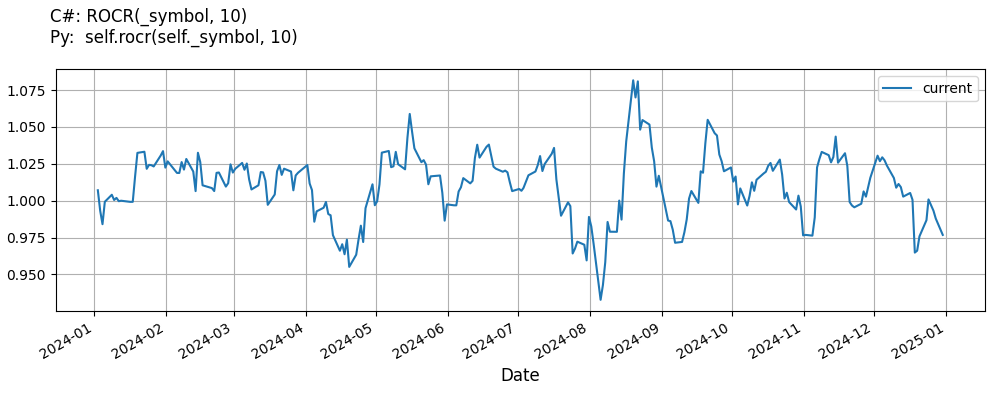# Supported Indicators

## Rate Of Change Ratio

### Introduction

This indicator computes the Rate Of Change Ratio (ROCR). The Rate Of Change Ratio is calculated with the following formula: ROCR = price / prevPrice

To view the implementation of this indicator, see the LEAN GitHub repository.

### Using ROCR Indicator

To create an automatic indicators for RateOfChangeRatio, call the ROCR helper method from the QCAlgorithm class. The ROCR method creates a RateOfChangeRatio object, hooks it up for automatic updates, and returns it so you can used it in your algorithm. In most cases, you should call the helper method in the Initialize method.

public class RateOfChangeRatioAlgorithm : QCAlgorithm
{
private Symbol _symbol;
private RateOfChangeRatio _rocr;

public override void Initialize()
{
_rocr = ROCR(_symbol, 10);
}

public override void OnData(Slice data)
{
{
// The current value of _rocr is represented by itself (_rocr)
// or _rocr.Current.Value
Plot("RateOfChangeRatio", "rocr", _rocr);

}
}
}
class RateOfChangeRatioAlgorithm(QCAlgorithm):
def Initialize(self) -> None:
self.rocr = self.ROCR(self.symbol, 10)

def OnData(self, slice: Slice) -> None:
# The current value of self.rocr is represented by self.rocr.Current.Value
self.Plot("RateOfChangeRatio", "rocr", self.rocr.Current.Value)



The following reference table describes the ROCR method:

### ROCR()1/1

            RateOfChangeRatio QuantConnect.Algorithm.QCAlgorithm.ROCR (
Symbol                           symbol,
Int32                            period,
*Nullable<Resolution>      resolution,
*Func<IBaseData, Decimal>  selector
)


Creates a new RateOfChangeRatio indicator.

If you don't provide a resolution, it defaults to the security resolution. If you provide a resolution, it must be greater than or equal to the resolution of the security. For instance, if you subscribe to hourly data for a security, you should update its indicator with data that spans 1 hour or longer.

You can manually create a RateOfChangeRatio indicator, so it doesn't automatically update. Manual indicators let you update their values with any data you choose.

Updating your indicator manually enables you to control when the indicator is updated and what data you use to update it. To manually update the indicator, call the Update method with time/number pair, or an IndicatorDataPoint. The indicator will only be ready after you prime it with enough data.

public class RateOfChangeRatioAlgorithm : QCAlgorithm
{
private Symbol _symbol;
private RateOfChangeRatio _rocr;

public override void Initialize()
{
_rocr = new RateOfChangeRatio(10);
}

public override void OnData(Slice data)
{
if (data.Bars.TryGeValue(_symbol, out var bar))
{
_rocr.Update(bar.EndTime, bar.Close);
}

{
// The current value of _rocr is represented by itself (_rocr)
// or _rocr.Current.Value
Plot("RateOfChangeRatio", "rocr", _rocr);

}
}
}
class RateOfChangeRatioAlgorithm(QCAlgorithm):
def Initialize(self) -> None:
self.rocr = RateOfChangeRatio(10)

def OnData(self, slice: Slice) -> None:
bar = slice.Bars.get(self.symbol)
if bar:
self.rocr.Update(bar.EndTime, bar.Close)

# The current value of self.rocr is represented by self.rocr.Current.Value
self.Plot("RateOfChangeRatio", "rocr", self.rocr.Current.Value)



To register a manual indicator for automatic updates with the security data, call the RegisterIndicator method.

public class RateOfChangeRatioAlgorithm : QCAlgorithm
{
private Symbol _symbol;
private RateOfChangeRatio _rocr;

public override void Initialize()
{
_rocr = new RateOfChangeRatio(10);
RegisterIndicator(_symbol, _rocr, Resolution.Daily);
}

public override void OnData(Slice data)
{
{
// The current value of _rocr is represented by itself (_rocr)
// or _rocr.Current.Value
Plot("RateOfChangeRatio", "rocr", _rocr);

}
}
}
class RateOfChangeRatioAlgorithm(QCAlgorithm):
def Initialize(self) -> None:
self.rocr = RateOfChangeRatio(10)
self.RegisterIndicator(self.symbol, self.rocr, Resolution.Daily)

def OnData(self, slice: Slice) -> None:
# The current value of self.rocr is represented by self.rocr.Current.Value
self.Plot("RateOfChangeRatio", "rocr", self.rocr.Current.Value)



The following reference table describes the RateOfChangeRatio constructor:

### RateOfChangeRatio()1/2

            RateOfChangeRatio QuantConnect.Indicators.RateOfChangeRatio (
string  name,
int     period
)


Initializes a new instance of the RateOfChangeRatio class using the specified name and period.

### RateOfChangeRatio()2/2

            RateOfChangeRatio QuantConnect.Indicators.RateOfChangeRatio (
int  period
)


Initializes a new instance of the RateOfChangeRatio class using the specified period.

### Visualization

The following image shows plot values of selected properties of RateOfChangeRatio using the plotly library.You can also see our Videos. You can also get in touch with us via Discord.